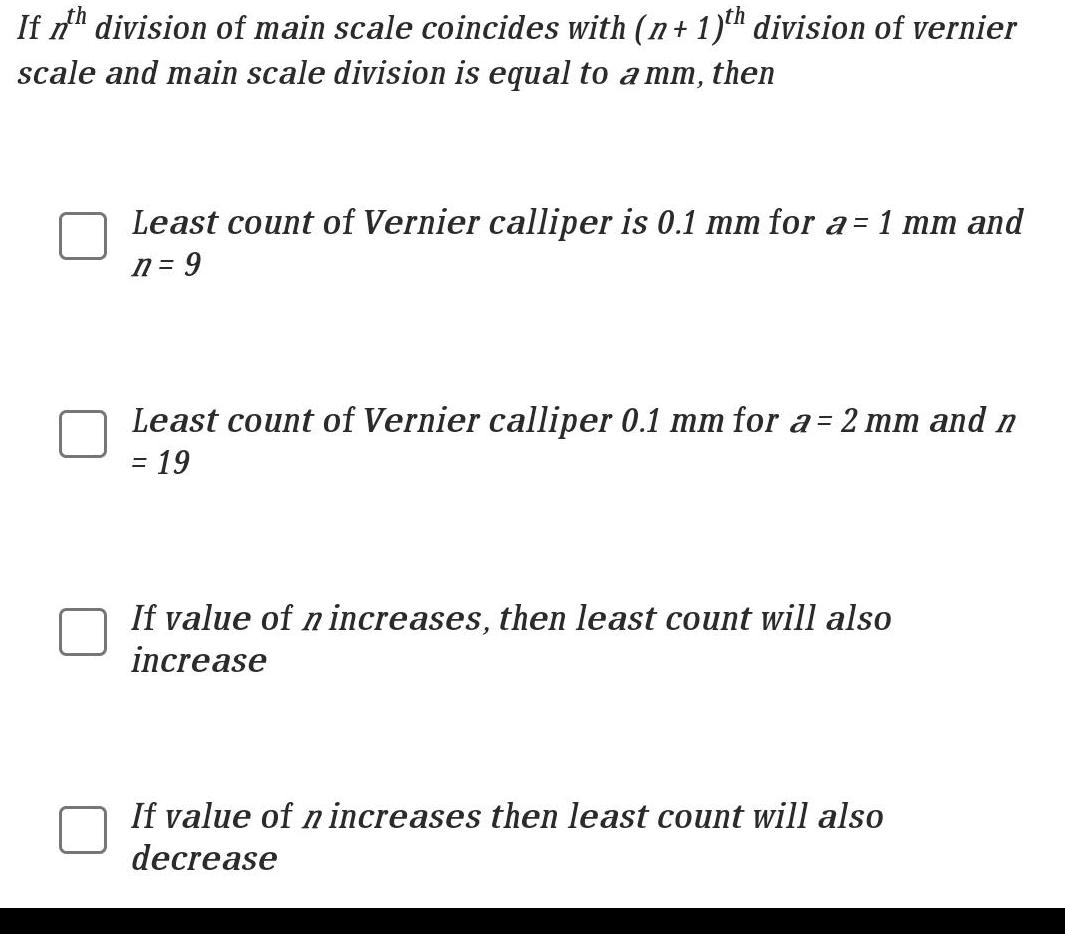Question:

# If nºh division of main scale coincides with (n+1)th

Last updated: 7/31/2022If nºh division of main scale coincides with (n+1)th division of vernier scale and main scale division is equal to a mm, then Least count of Vernier calliper is 0.1 mm for a = 1 mm and n = 9 Least count of Vernier calliper 0.1 mm for a= 2 mm and n = 19 If value of n increases, then least count will also increase If value of n increases then least count will also decrease